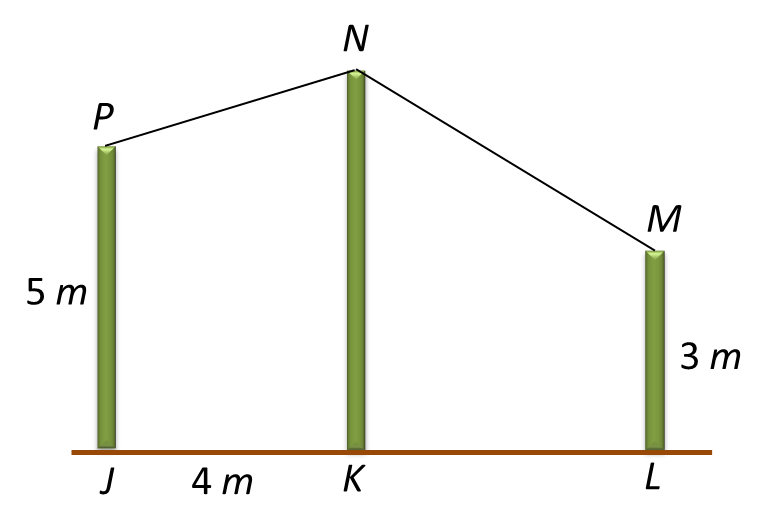# 10.3.2 Angles of Elevation and Depression, Paper 1 (Short Questions)

Question 4:
Diagram below shows three vertical poles JP, KN and LM, on a horizontal plane.The angle of elevation of N from P is 15o.
The angle of depression of M from N is 35o.
Calculate the distance, in m, from K to L.

Solution:$\begin{array}{l}\mathrm{tan}\angle APN=\frac{AN}{PA}\\ \mathrm{tan}{15}^{o}=\frac{AN}{4}\\ AN=4×0.268\\ AN=1.072m\\ \text{Length of}BN=2+1.072=3.072cm\\ \\ \mathrm{tan}\angle BMN=\frac{BN}{BM}\\ \mathrm{tan}{35}^{o}=\frac{3.072}{BM}\\ BM=\frac{3.072}{0.700}=4.389\\ \text{Distance of}KL=4.389m\end{array}$

Question 5:
Diagram below shows two vertical poles JM and LN, on a horizontal plane.The angle of elevation of M from K is 70oand the angle of depression of K from N is 40o.
Find the difference in distance, in m, between JK and KL.

Solution:$\begin{array}{l}\mathrm{tan}\angle JKM=\frac{14}{JK}\\ JK=\frac{14}{\mathrm{tan}{70}^{o}}\\ JK=5.096m\\ \\ \mathrm{tan}\angle LKN=\frac{8}{KL}\\ KL=\frac{8}{\mathrm{tan}{40}^{o}}\\ KL=9.534m\\ \\ \text{Difference in distance of}JK\text{and}KL\\ =9.534-5.096\\ =4.438m\end{array}$